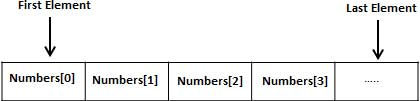# Pascal - Arrays

#### Complete Python Prime Pack

9 Courses     2 eBooks

#### Artificial Intelligence & Machine Learning Prime Pack

6 Courses     1 eBooks

#### Java Prime Pack

9 Courses     2 eBooks

Pascal programming language provides a data structure called the array, which can store a fixed-size sequential collection of elements of the same type. An array is used to store a collection of data, but it is often more useful to think of an array as a collection of variables of the same type.

Instead of declaring individual variables, such as number1, number2, ..., and number100, you declare one array variable such as numbers and use numbers, numbers, and ..., numbers to represent individual variables. A specific element in an array is accessed by an index.

All arrays consist of contiguous memory locations. The lowest address corresponds to the first element and the highest address to the last element.

Please note that if you want a C style array starting from index 0, you just need to start the index from 0, instead of 1.## Declaring Arrays

To declare an array in Pascal, a programmer may either declare the type and then create variables of that array or directly declare the array variable.

The general form of type declaration of one-dimensional array is −

```type
array-identifier = array[index-type] of element-type;
```

Where,

• array-identifier − indicates the name of the array type.

• index-type − specifies the subscript of the array; it can be any scalar data type except real

• element-type − specifies the types of values that are going to be stored

For example,

```type
vector = array [ 1..25] of real;
var
velocity: vector;
```

Now, velocity is a variable array of vector type, which is sufficient to hold up to 25 real numbers.

To start the array from 0 index, the declaration would be −

```type
vector = array [ 0..24] of real;
var
velocity: vector;
```

## Types of Array Subscript

In Pascal, an array subscript could be of any scalar type like, integer, Boolean, enumerated or subrange, except real. Array subscripts could have negative values too.

For example,

```type
temperature = array [-10 .. 50] of real;
var
day_temp, night_temp: temperature;
```

Let us take up another example where the subscript is of character type −

```type
ch_array = array[char] of 1..26;
var
alphabet: ch_array;
```

Subscript could be of enumerated type −

```type
color = ( red, black, blue, silver, beige);
car_color = array of [color] of boolean;
var
car_body: car_color;
```

## Initializing Arrays

In Pascal, arrays are initialized through assignment, either by specifying a particular subscript or using a for-do loop.

For example −

```type
ch_array = array[char] of 1..26;
var
alphabet: ch_array;
c: char;

begin
...
for c:= 'A' to 'Z' do
alphabet[c] := ord[m];
(* the ord() function returns the ordinal values *)
```

## Accessing Array Elements

An element is accessed by indexing the array name. This is done by placing the index of the element within square brackets after the name of the array. For example −

```a: integer;
a: = alphabet['A'];
```

The above statement will take the first element from the array named alphabet and assign the value to the variable a.

Following is an example, which will use all the above-mentioned three concepts viz. declaration, assignment and accessing arrays −

```program exArrays;
var
n: array [1..10] of integer;   (* n is an array of 10 integers *)
i, j: integer;

begin
(* initialize elements of array n to 0 *)
for i := 1 to 10 do
n[ i ] := i + 100;   (* set element at location i to i + 100 *)
(* output each array element's value *)

for j:= 1 to 10 do
writeln('Element[', j, '] = ', n[j] );
end.
```

When the above code is compiled and executed, it produces the following result −

```Element = 101
Element = 102
Element = 103
Element = 104
Element = 105
Element = 106
Element = 107
Element = 108
Element = 109
Element = 110
```

## Pascal Arrays in Detail

Arrays are important to Pascal and should need lots of more details. There are following few important concepts related to array which should be clear to a Pascal programmer −

Sr.No Concept & Description
1 Multi-dimensional arrays

Pascal supports multidimensional arrays. The simplest form of the multidimensional array is the two-dimensional array.

2 Dynamic array

In this type of arrays, the initial length is zero. The actual length of the array must be set with the standard SetLength function.

3 Packed array

These arrays are bit-packed, i.e., each character or truth values are stored in consecutive bytes instead of using one storage unit, usually a word (4 bytes or more).

4 Passing arrays to subprograms

You can pass to a subprogram a pointer to an array by specifying the array's name without an index.